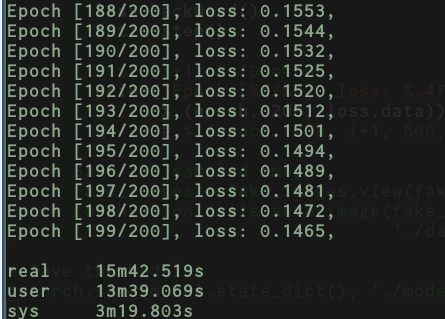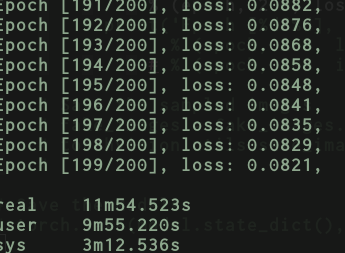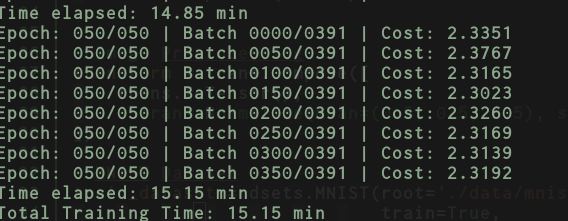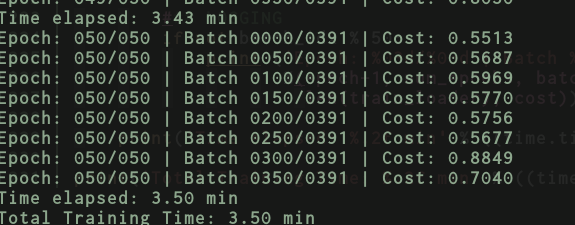Hi folks,
I’ve noticed a couple of things going on with DataLoader.

1. Transofrms are only invoked when train_loader iterator is invoked in the training loop. This is quite expensive. I dunno if this is intended behavriour?
``````# Image Preprocessing
transform = trans.Compose([
trans.ToTensor(),
trans.Normalize(mean=(0.5, 0.5, 0.5), std=(0.5, 0.5, 0.5)),
])

# MNIST Dataset
train_dataset = dsets.MNIST(root='./data/mnist/',
train=True,
transform=transform,

dataset=train_dataset,
batch_size=train_dataset.train_data.shape,
shuffle=True,
num_workers=10,
pin_memory=True
)

import pdb; pdb.set_trace()
# Now examine the train_dataset.train_data
``````
1. The `train_dataset.train_data` is scaled between [-1, +1] shouldn’t that be between [0, 1]. Since the scaling happens automatically via `ToTensor()` invocation how can we tell `ToTensor()` to scale between [0, 1]?

2. This might not be correct but here’s the training example trying to use data loader to perform full batch:

``````for epoch in range(200):
for i, (images, labels) in enumerate(train_loader):
# Build mini-batch dataset
images = images.cuda(1)
labels = labels.cuda(1)
images = images.view(images.size(0), -1)
``````

Using this approach of data loader + full batch training I get the following speeds:1. If I bypass completely the data loader and do the full batch training on my own here’s what I get:Am I doing sth fundamentally wrong here?

Maybe It would be nice for the data loader to have flag indicating to load all data in memory if possible?

2 Likes
1. All transformations are performed on the fly while loading the next batch. Using multiprocessing (`num_workers>0` in your `DataLoader`) you can load and process your data while your GPU is still busy training your model, thus possibly hiding the loading and processing time of your data.

2. `ToTensor()` will scale your data to `[0, 1]`. Since you apply `Normalize(mean=(0.5, 0.5, 0.5), std=(0.5, 0.5, 0.5))` afterwards, you’ll get the new range `[-1, 1]`.

3. I guess by “full batch”, you’ve specified the `batch_size=60000`?

4. In fact, MNIST is so small that all data is already loaded into RAM. You can get the data using `dataset.data`. Did you push all your data samples to the GPU beforehand?

1 Like

Same behaviour even with `num_workers=0 or 1`

Oops, good catch, just missed that!

Yup!

That happens inside the epochs loop. Is that bad?

Besides I don’t see any of the above increasing so much the time of DataLoader. I would have expected using DataLoader and batch size 60000 to be equivalent in terms of speed with my own testing when I’m not using the DataLoader but the full dataset? It’s hard that this would happen by only by the answer you mentioned on point 1).

The current implementation uses a single worker to load a batch. Since you specified the batch size as the complete dataset, the number of workers won’t change anything in this edge case. There is a feature request for using multiple workers loading a single batch, which would yield a speedup in your case.

You will add a lot of overhead, since the batch is created by calling the `Dataset`'s `__getitem__` method for each sample, i.e. 60000 times in your case.

Did you apply the same transformations in the second case? Also, did you shuffle the data somehow?
Could you share your code to compare both approaches? The current difference is huge, so that I would like to debug it a bit further.

That was missing in the first comparison.
Now with same transformations and very naive shuffling on full batch:Sure thing, the code below runs full-batch, if you un-comment the commented lines and then it should run using DataLoader. When running with DataLoader comment the lines above the for loop for images, labels etc.:

``````import torch
import torch.nn as nn
import torch.nn.functional as F
import torchvision.datasets as dsets
import torchvision.transforms as trans

# Image Preprocessing
transform = trans.Compose([
trans.ToTensor(),
])

# MNIST Dataset
train_dataset = dsets.MNIST(root='./data/mnist/',
train=True,
transform=transform,

dataset=train_dataset,
batch_size=train_dataset.train_data.shape,
shuffle=True,
num_workers=10,
pin_memory=True
)

# MLP
class MLP(nn.Module):
def __init__(self):
super(MLP, self).__init__()
self.fc1 = nn.Linear(784, 512)
self.fc2 = nn.Linear(512, 256)
self.fc3 = nn.Linear(256, 128)
self.fc4 = nn.Linear(128, 10)

def forward(self, x):
h = F.relu(self.fc1(x))
h = F.relu(self.fc2(h))
h = F.relu(self.fc3(h))
out = F.log_softmax(self.fc4(h), dim=-1)
return out

model = MLP()
# criterion = nn.CrossEntropyLoss()
criterion = nn.NLLLoss()
model.cuda(1)

train_dataset.train_data = train_dataset.train_data.float().div(255.0)
images = train_dataset.train_data.cuda(1)
labels = train_dataset.train_labels.cuda(1)

# Training
for epoch in range(200):
# for i, (images, labels) in enumerate(train_loader):
# Build mini-batch dataset
# images = images.cuda(1)
# labels = labels.cuda(1)
images = images.view(images.size(0), -1)
temp = torch.cat([
images,
labels.view(images.size(0), 1).to(torch.float)
], dim=1)
idx = torch.randperm(temp.nelement())
temp.view(-1)[idx].view(temp.size())
images = temp[:, :images.size(1)]
labels = temp[:, -1].to(torch.long)

# Train the model
outputs = model(images)
loss = criterion(outputs, labels)

loss.backward()
optim.step()

print('Epoch [%d/%d], loss: %.4f,'
% (epoch, 200, loss.data))``````

Is there a more efficient and better way to do shuffling than my naive way? Essentially is there anything equivalent to `numpy.random.shuffle()` in pytorch?

Transofrms are only invoked when train_loader iterator is invoked in the training loop. This is quite expensive. I dunno if this is intended behavriour?

When do you want to invoke it otherwise? Before iterating? you can do that, but then you have to store a seperate copy of your image dataset (considering that you don’t want to modify your original images because you might want to try other transformations in other projects)

Also, the data transformation is so fast that it really doesn’t cause any bottleneck on typical dataset if you have num_workers>=4 (I benchmarked this in the past)

The train_dataset.train_data is scaled between [-1, +1] shouldn’t that be between [0, 1]. Since the scaling happens automatically via ToTensor() invocation how can we tell ToTensor() to scale between [0, 1]?

If you want [0, 1] range, simply drop the

`trans.Normalize(mean=(0.5, 0.5, 0.5), std=(0.5, 0.5, 0.5)),`

Maybe It would be nice for the data loader to have flag indicating to load all data in memory if possible?

I think this would kill the purpose of the data “loader” In that case, simply load the dataset into memory and shuffle before each epoch

Is there a more efficient and better way to do shuffling than my naive way? Essentially is there anything equivalent to `numpy.random.shuffle()` in pytorch?

`````` all_your_images = torch.randn(500, 3, 28, 28)
shuffleimages =  all_your_images[torch.randperm(500)]
``````

It doesn’t seem like the case here. I mean comparing DataLoader using full batch with simply full batch in my case without the DataLoader, in both cases same transformations. Still full batch without DataLoader is almost half the time that it takes the DataLoader with full batch, and here is where I don’t understand why so much discrepancy?

Thanks this is what I’m already doing:

I dunno if there’s a more efficient way?

The speed difference is because you are loading the whole dataset from disk. Also, naturally, the larger the dataset the longer the loading time. In a real-world scenario more than one batch will usually loaded before the network finishes the the forward and backward loop. To make your benchmark fair,

a) use a dataloader with a reasonable batchsize and reasonable number of workers and train the network

b) compare it with a network where your dataset is in memory like right now, but iterate over it with the same batch size.

Cat is naturally very expensive. Just keep an index over all your images that you shuffle and then use it as a mask instead of making new arrays each time. The number of images doesn’t change, so you can simply reuse (reshuffle) that index instead of creating new arrays each time.

PS: I see that you are using 10 workers. That is probably too much and will slow you down due to the communication overhead between processes. I think 4 is a reasonable number because the dataset is rel small.Oh wow, that’s quite some difference. How large is your neural net approx? And are you transferring the data onto the GPU during training?

Another Q: are you using an SSD or an HD?

4 - Dense layers

This might have been sth that I missed since I transferred them once before the training loop. Let me re-run this.

I am using SSD

Transferring every mini-batch on gpu during the training loop:Hm, this weird. I suspect you may have another hardware-related bottleneck somewhere then, maybe the CPU!? I just tested it on my machine and get the same speed when loading the dataset via the dataloader versus iterating over the dataset stored in memory. For simplicity, here, I used a vanilla VGG16 model and CIFAR10. I uploaded the code as Gists if you want to test it on your machine – they are both standalone.

the vgg16.py file uses a dataloader with batchsize 128 and 4 workers. The vgg16_inmem.py loads the CIFAR10 dataset into memory and then shuffles it before every epoch before iterating over the minibatches.

Timing-wise, I get for vgg16.py:

``````Epoch: 001/050 | Batch 0000/0391 | Cost: 302.2942
Epoch: 001/050 | Batch 0050/0391 | Cost: 2.3336
Epoch: 001/050 | Batch 0100/0391 | Cost: 2.1539
Epoch: 001/050 | Batch 0150/0391 | Cost: 2.0014
Epoch: 001/050 | Batch 0200/0391 | Cost: 1.9107
Epoch: 001/050 | Batch 0250/0391 | Cost: 1.8304
Epoch: 001/050 | Batch 0300/0391 | Cost: 1.9485
Epoch: 001/050 | Batch 0350/0391 | Cost: 1.5591
Time elapsed: 0.23 min
...
Epoch: 050/050 | Batch 0000/0391 | Cost: 0.1005
Epoch: 050/050 | Batch 0050/0391 | Cost: 0.1097
Epoch: 050/050 | Batch 0100/0391 | Cost: 0.0284
Epoch: 050/050 | Batch 0150/0391 | Cost: 0.1244
Epoch: 050/050 | Batch 0200/0391 | Cost: 0.0822
Epoch: 050/050 | Batch 0250/0391 | Cost: 0.0908
Epoch: 050/050 | Batch 0300/0391 | Cost: 0.1248
Epoch: 050/050 | Batch 0350/0391 | Cost: 0.0926
Time elapsed: 11.68 min
``````

And for vgg16_inmem.py:

``````Epoch: 001/050 | Batch 0000/0391 | Cost: 331.2111
Epoch: 001/050 | Batch 0050/0391 | Cost: 2.3051
Epoch: 001/050 | Batch 0100/0391 | Cost: 2.1995
Epoch: 001/050 | Batch 0150/0391 | Cost: 2.0644
Epoch: 001/050 | Batch 0200/0391 | Cost: 1.8679
Epoch: 001/050 | Batch 0250/0391 | Cost: 1.7329
Epoch: 001/050 | Batch 0300/0391 | Cost: 1.8466
Epoch: 001/050 | Batch 0350/0391 | Cost: 1.8419
Time elapsed: 0.23 min
...
Epoch: 050/050 | Batch 0000/0391 | Cost: 2.3039
Epoch: 050/050 | Batch 0050/0391 | Cost: 2.3029
Epoch: 050/050 | Batch 0100/0391 | Cost: 2.3163
Epoch: 050/050 | Batch 0150/0391 | Cost: 2.3132
Epoch: 050/050 | Batch 0200/0391 | Cost: 2.3173
Epoch: 050/050 | Batch 0250/0391 | Cost: 2.3053
Epoch: 050/050 | Batch 0300/0391 | Cost: 2.3009
Epoch: 050/050 | Batch 0350/0391 | Cost: 2.3057
Time elapsed: 11.61 min
``````

So, on my machine, there does not seem to be any benefit in loading data in memory versus using the DataLoader. Might be different though on different machines if the CPU and drive are not fast enough to load the next batch for the GPU.

Thanks Sebastian,

Here’s what I’ve noticed.
For big models like vgg and others probably the difference between DataLoader and No DataLoader is negligible.

Here are the results for `vgg16.py` on TitanXAnd for `vgg16_inmemory.py`But if I swap your vgg with just the small model I had before with just a couple of dense layers then, the results for `vgg16_inmemory.py` --> now just contains a couple of dense layers only.And the results for `vgg16.py` --> now just a couple of dense layers.Fair point. Just replaced that VGG16 with a network with only two fully connected layers and the data loader was indeed slow. In that case the reading from disk via PIL may have been the limiting factor. But when I crank up the number of workers to an insane 50, I get much closer to the in-memory variant.

In any case, in a practical use case scenario, if your data set is small and fits into memory, why not using the dataloader to fetch data from an array in memory instead of from disk?

Thanks for the suggestion, do you have any pointers to examples. My understanding was that when you create the dataset you’ll have to specify the `root` where the data is. Usually that points to a directory on disk could that also be just numpy array?

``````train_dataset = dsets.MNIST(root=numpy.ranom.randn(100, 784), train=True, transform=transform, download=False)
``````

Oh i see. That’s nothing to do with the DataLoader but that’s just the case for these pre-bundled datasets. You can create a dataset however you like, it doesn’t have to be for loading from a directory on your drive. E.g., here is a Dataset example for CelebA images:

``````class CelebaDataset(Dataset):

def __init__(self, txt_path, img_dir, transform=None):

df = pd.read_csv(txt_path, sep=" ", index_col=0)
self.img_dir = img_dir
self.txt_path = txt_path
self.img_names = df.index.values
self.y = df['Male'].values
self.transform = transform

def __getitem__(self, index):
img = Image.open(os.path.join(self.img_dir,
self.img_names[index]))

if self.transform is not None:
img = self.transform(img)

label = self.y[index]
return img, label

def __len__(self):
return self.y.shape
``````

In other words, it’s loading from disk because we ask it to :). But you can simply define the getitem method to yield a row from your data tensor held in memory.

(I have the full example for the code snippet above here: https://github.com/rasbt/deep-learning-book/blob/master/code/model_zoo/pytorch_ipynb/custom-data-loader-celeba.ipynb)

Oh I see. Just like building your own custom generator.
Thanks Sebastian!

Yes, the DataLoader is more like a thin layer to facilitate minibatching with shuffling etc. It’s particularly nice because it’s so easy to use and not overly complicated.

1 Like